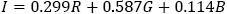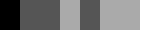# Image Processing Algorithms Part 3: Greyscale Conversion

This article was originally published in issue 54 of The Crypt Mag

In this article we will look at how to convert a colour image to greyscale.

In order to convert a particular colour into greyscale we need to work out what the intensity or brightness of that colour is. A quick way of calculating the intensity is to obtain the mean value of the red, green and blue components. The formula for this is:Although it can produce reasonable results, this method is not perfect. The reason for this is that the human eye does not perceive reds, greens and blues at the same intensity level. The colour green, for example, at maximum intensity looks brighter than blue at maximum intensity.

Another method of greyscale conversion which takes into account the human perception of colour uses different weights for the red, green and blue components. This means that when you are calculating the value for the intensity each of the colour components are multiplied by a weight value. There are a number of different values used for these weights but one of the most popular is the following:To illustrate the above points let’s have a look at an image of some coloured bars:Let’s convert this image to greyscale using the mean method:The intensity levels are not right here as there should be a gradual change in intensity going from the black to the white.

Converting the image to greyscale using the weighted method will give us the following:As you can see the weighted conversion is a much more accurate representation of the original coloured image than the mean conversion.

Let’s have a look at the ‘Lena’ and ‘Mandrill’ images again which have been converted to greyscale using both methods:

Although not accurate, the mean conversion does produce reasonable results in these cases. Closer inspection between the mean and weighted conversions will reveal that some sections of the image converted using the mean conversion method appear slightly darker or slightly lighter than they should be.